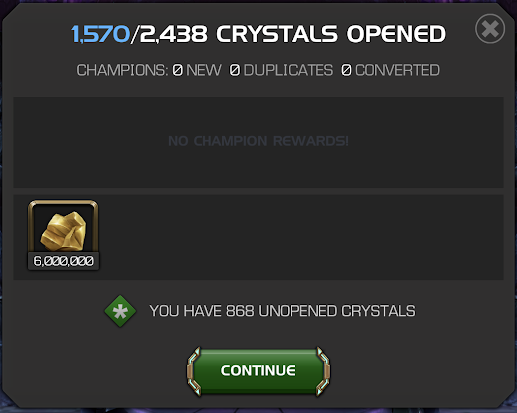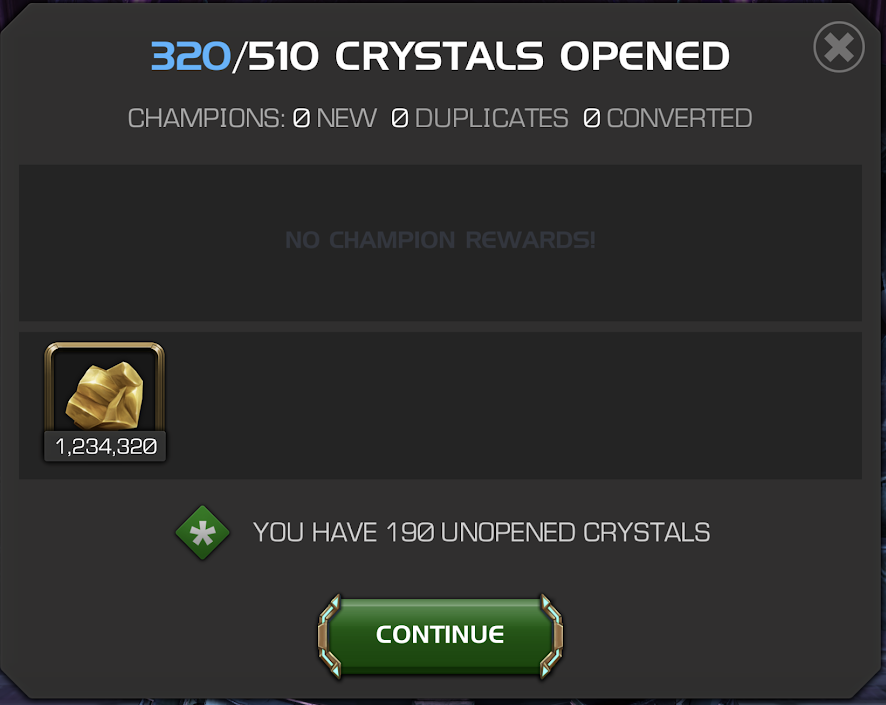# Gold crystal drop odds analysis (with data speed up trick)

For those that just want the estimated drop odds for the gold crystal, here they are (the value in parenthesis is with the 20% gold boost active).

76% 2400 (2880)
21% 5000 (6000)
3% 10000 (12000)

Average gold per crystal: 3174

Here is the tabulated data for 2930 gold crystals with calculated percentages:

12000 gold - 93 - 3.174%
6000 gold - 613 - 20.92%
2880 gold - 2224 - 75.90%

Margin for error: about one percentage point for the top two values, less than half a percentage point for the last one. So the 3% is fairly certain, the other numbers are in the general range of 20:77 and 22:75.

The interesting question is how I gathered that much data. It would have been daunting to open 2930 crystals one at a time, like I did for arena crystals (see: Arena crystal drop odds for my post on measuring the drop odds for normal and uncollected arena crystals). Turns out there's a short cut that works for gold crystals. I don't think this will work for arena crystals. Those not interested in this kind of data gathering or analysis can skip the rest of the post.

Suppose you were to open ten gold crystals, and you ended up with 44160 gold. What did you pull? As it turns out, there's only one possibility: those ten crystals were 7 that opened for 2880, 2 that opened 6000, and 1 that opened for 12000 gold. There's no other possibility. And the reason is because the lowest option is not divisible by 1000, but the other two options are. There are only two possible ways to end up with XXX60 gold: you opened two crystals for 2880 and the rest were higher, or you opened seven crystals for 2880 and the rest were higher openings. That's because 2 x 80 = 160 and 7 x 80 = 560. That's the only two ways to get the "60." Furthermore, there's only one way to get "160" and that's 7 x 2880 = 20160. For every value of the last three digits there's only one way to get that number with N x 2880, and the other two drops can't help you (they all end in 000).

[For the mathematically inclined: the technical property here is that the least common multiple of 880 and 1000 is 22000, which means Nx880 mod 1000 doesn't repeat for N<=10]

So the gold amount actually "encodes" how many 2880 drops you got. 880 = 1, 760 = 2, 640 = 3, 520 = 4, 400 = 5, 280 = 6, 160 = 7, 040 = 8, and 920 = 9. If you subtract that from the original total, you end up with a number that is always divisible by 6000 (because the other two drops are both divisible by 6000). It isn't hard from there to figure out what the other two amounts are: in this case you have three unaccounted for drops and 44160 - 7x2880 = 24000 gold left. That's two drops of 6000 and one drop of 12000. There is always only one possible solution, because this is a set of two linear equations with two variables: 6000x + 12000y = 24000; x+y = 3. Basic algebra states if this set of equations has any solutions it has exactly one such solution. And since all other drop options will just change the constants, all possibilities will have exactly one solution.

So if you open ten gold crystals and note the amount of gold you get, you can directly determine what all ten drops were. So all you do is open ten gold crystals at a time and note the running total of gold in a table. Then you simply subtract the current value from the previous value to get the amount of gold for those ten crystals. In this way, you can open thousands of crystals ten at a time, just writing down one number after each pop. Later you can go back and do the subtraction to get the gold for each ten pop, and then determine the ten drops, and then sum up across all pops.

That's how I was able to generate that much data in a relatively short amount of time. Anyone could do this relatively quickly, of course they would have had to save up the gold crystals to do it. And before anyone asks, I don't have enough uncollected gold crystals to estimate their drop odds with any accuracy. Although it is possible they follow these crystals, just with higher numbers.

How much data would it take to reduce the margin for error to be reasonably certain of the last digit of the top two (higher) percentage odds? Probably about four times the data, or about 12,000 crystals (i.e. 9,000 more). I'll see you all again in 2023.

If you've been reading up to here, maybe you're interested in why this doesn't work for arena crystals. Unfortunately it doesn't. But the reason why it doesn't does go more into the weeds mathematically, but I'll try to simplify. I think an example would demonstrate the problem quicker than the math. Suppose you were to get 450 units in a pop set of openings? What did you get? Well, there's a number of different possibilities: you could have gotten six 75 unit openings, or two 225 openings, or even three 75 and one 225. Now suppose you also got 150k gold. That also has a number of different possibilities, including 6 25k, 3 50k, or 2 25k and 2 50k. Now let's say you got both: 450 units and 150k gold. Granted this is unusual, but this just illustrates the point. In this scenario there are two indistinguishable possibilities: two 225 units and 6 25k gold in eight crystals, or three 75 and one 225 units and 2 25k gold and 2 50k gold in eight crystals. Both add up to the same total. So there isn't a unique solution here.

The problem here is you have two equations with four unknowns, so there are not guaranteed to be unique solutions in every case. As these are diophantine equations, there often is only one sensible solution, but the multiple solution situations make the data collection method dangerous. You could try to analyze for all possible non-unique solution environments and simply throw them out, but that would introduce a skew into the data. There are other ways to attempt to correct for this statistical problem, probably resorting to some form of stochastic computational analysis. This would require a ton more data. Basically, for arena crystals it is probably simpler, unfortunately, to open them one at a time.